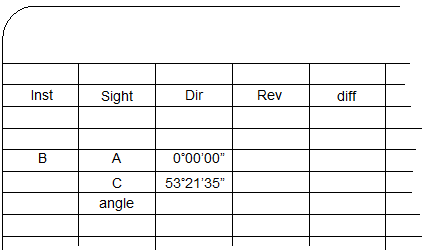## 3. Example measurement and field notesFigure D-6 Example Angle Measurement

We will show the process of measuring an angle and recording the readings. In our example, the TSI will occupy point B and measure the angle right (clockwise) from A to C, Figure D-6.

There are various note formats which can be used depending on the project and other measurements being made. Running a traverse, for example, would use a format which incorporates distances as well as angles.

For this example, we will use a simple angle only format.

The procedure and notes are shown in Figure D-7.

 Set up the column headings. Direct and Reverse angle readings are recorded in columns. A D/R angle set flows across the pageThe TSI is set on point B and carefully sighted on point A. The angle is set to zero and recorded in the Dir column for point A.The lock is released and TSI rotated in the direction of the angle to point C. In this case,the direction is to the right. Using the lock and slow motion, point C is accurately sighted. The angle reading is frozen, read, and recorded for point C in the Dir column.The value of the angle is the difference between the FS and BS readings. It should be computed immediately after the FS reading is made. This is the direct angle.The telescope is reversed about the HA so it points away from C. Then the horizontal lock is loosened and instrument rotated to the right back to point A. Using the lock and slow motion, point A is accurately sighted. The angle reading is released, read, and recorded for point A in the Dir column. The angle reading to point A should be the same as the previous one to C. If the reading was released before final sighting on point A then the initial BS value will differ from the final FS value. Whatever it is, record the horizontal angle reading displayed before turning to point C.The lock is released and TSI rotated right to point C. Using the lock and slow motion, point C is accurately sighted. The angle reading is frozen, read, and recorded for point C in the Rev column.The value of the reverse angle is the difference between the FS and BS readings. The direct and reverse angle should be close. Their difference should be primarily due to instrumental and pointing errors.The process would be repeated for the number of D/R sets required. Successive direct and reverse angle sets should be relatively consistent taking into account random errors.If all the angles are within acceptable limits, the final angle is computed as the average of all the D/R angles.Figure D-7 Angle Measurement Process and Notes